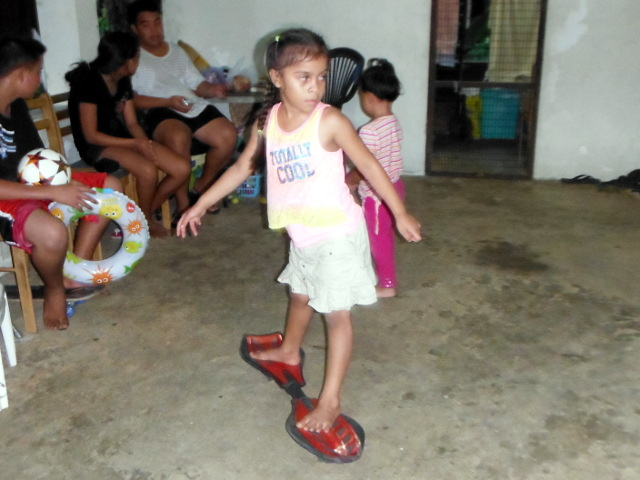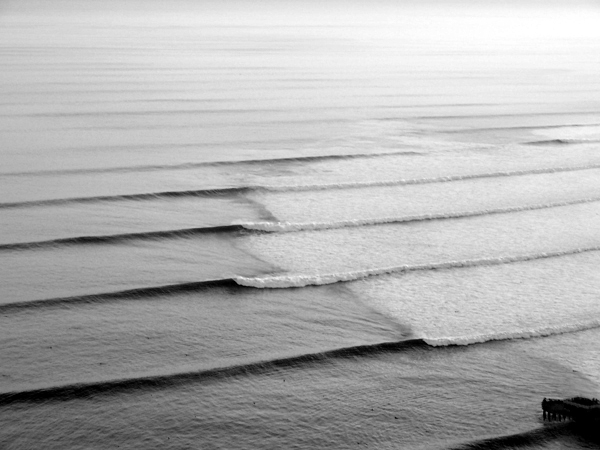psf3 094 ∿ 16 October 2015 Name:

1. A RipStik was swizzled ("wiggled") across a poster paper just as I did on Wednesday. The sinusoidal swizzle wave can be seen in the diagram.1. _________ How many waves are there on the "paper" above?
2. λ = _________ _________ What is the wavelength λ of ONE wave?
3. a = _________ _________ What is the amplitude a?
4. τ = _________ _________ What is the period τ of ONE wave?
5. f = _________ _________ Calculate the RipStik swizzle wave frequency f.
6. ѵwave = _________ _________ Use the wavelength λ and frequency f to calculate the velocity ѵwave of the RipStik swizzle wave.
2. Sketch a wave with a wavelength of 12 centimeters and an amplitude of 2 centimeters for x = 0 to 12 centimeters
3. During the laboratory the west wind rose up the ridge to the east of campus generating rain as the air rose up the ridgeline. This is called orographic or terrain induced rain. Explain how the wind and the ridge produce this rain.
4. A student gathered the data seen in the table below.
1. Plot the data on the graph.
2. __________ __________ Calculate the experimental speed of sound based on the data. Include the units with your answer.
3. __________The published value was 349 m/s. Calculate the percentage error for this data.
4. __________ __________ Based on the experimental data, how long for a sound to travel 615.6 meters?
5. In the following image identify the crests and troughs.period τ = 1 ÷ (frequency f )
velocity ѵ = wavelength λ * frequency f
$\text{percent error}=\frac{\left(\text{experimental value}-\text{expected value}\right)}{\left(\text{expected value}\right)}$